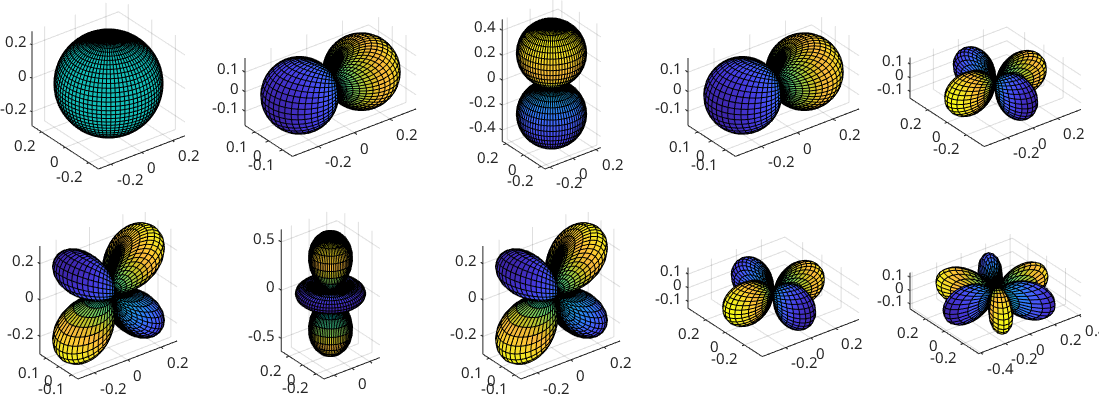Spherical Harmonics edit page

The spherical harmonics are special functions on the 2-sphere $$\mathbb S^2$$. In terms of polar coordinates $${\bf \xi} = (\sin \theta \cos \rho, \sin \theta \sin \rho, \cos \theta)$$ the spherical harmonic of degree $$m$$ and order $$l$$ is defined by

$Y_m^l({\bf \xi}) = \sqrt{\frac{2m+1}{4\pi}}P_m^{|l|}(\cos\rho)\mathrm e^{\mathrm i l\theta}$

where $$P_m^{|l|}$$, $$m \in {\bf N_0}$$, and $$l = -m, \ldots m$$ denote the associated Legendre-Polynomials.

We get the function values of the spherical harmonics of degree 1 in a point v by the command sphericalY, i.e.

The spherical harmonics form an orthonormal basis in $$L_2(\mathbb S^2)$$. Hence we describe functions on the 2-sphere by there harmonic representation using the class S2FunHarmonic.

With that we define the spherical harmonic $$Y_1^1$$ by

Various normalizations for the sperical harmonics are common in the literature.

Here we define the $$L_2$$-norm by

$\| f \|_2 = \left(\int_{\mathrm{sphere}} \lvert f(\xi)\rvert^2 \,\mathrm d\xi\right)^{1/2}$

such that $$\| 1 \|_2^2 = 1$$. Take a look on the section Integration of S2Fun's.

Using that definition the spherical harmonics in MTEX fulfill

$\| Y_m^l \|_2 = 1$ for all $$m,l$$.

To concluse this section we plot the first ten spherical harmonics